# Problems of the Week

Contribute a problem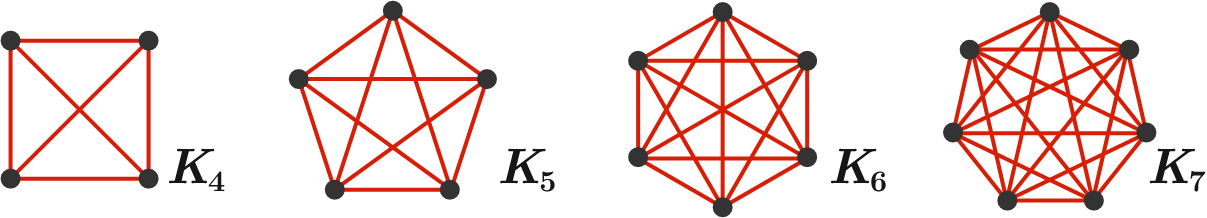In the graphs above, only $K_4$ can be drawn on a plane without any edge crossings, as illustrated below: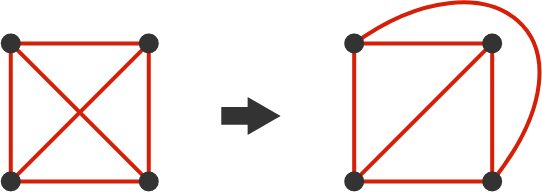This cannot be done with $K_5$, yet it can be drawn on a torus without any edge crossings: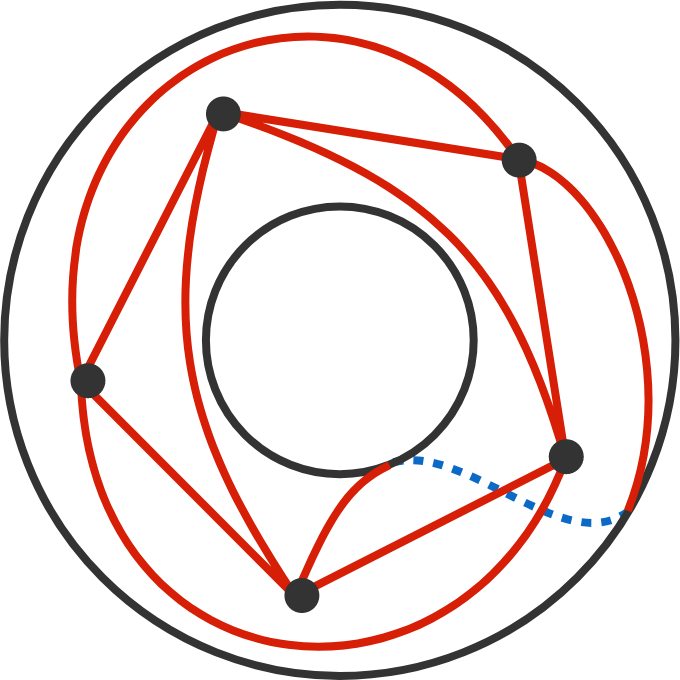Top view of the torus. The blue dotted line is on the bottom side.

Can $K_6$ and $K_7$ be drawn on a torus without edge crossings?

Agatha is at point $A,$ and needs to reach her son Damien at point $D$ as soon as possible. She can swim at a constant rate, and she can also run along the shore at a constant rate (her swimming and running speeds are different).

The diagram shows how long each segment takes her to travel. A direct route $A \rightarrow D$ takes 61 s, but so does a route of $A \rightarrow B \rightarrow C \rightarrow D.$

Find the minimum possible amount of time (in seconds) required for Agatha to reach Damien.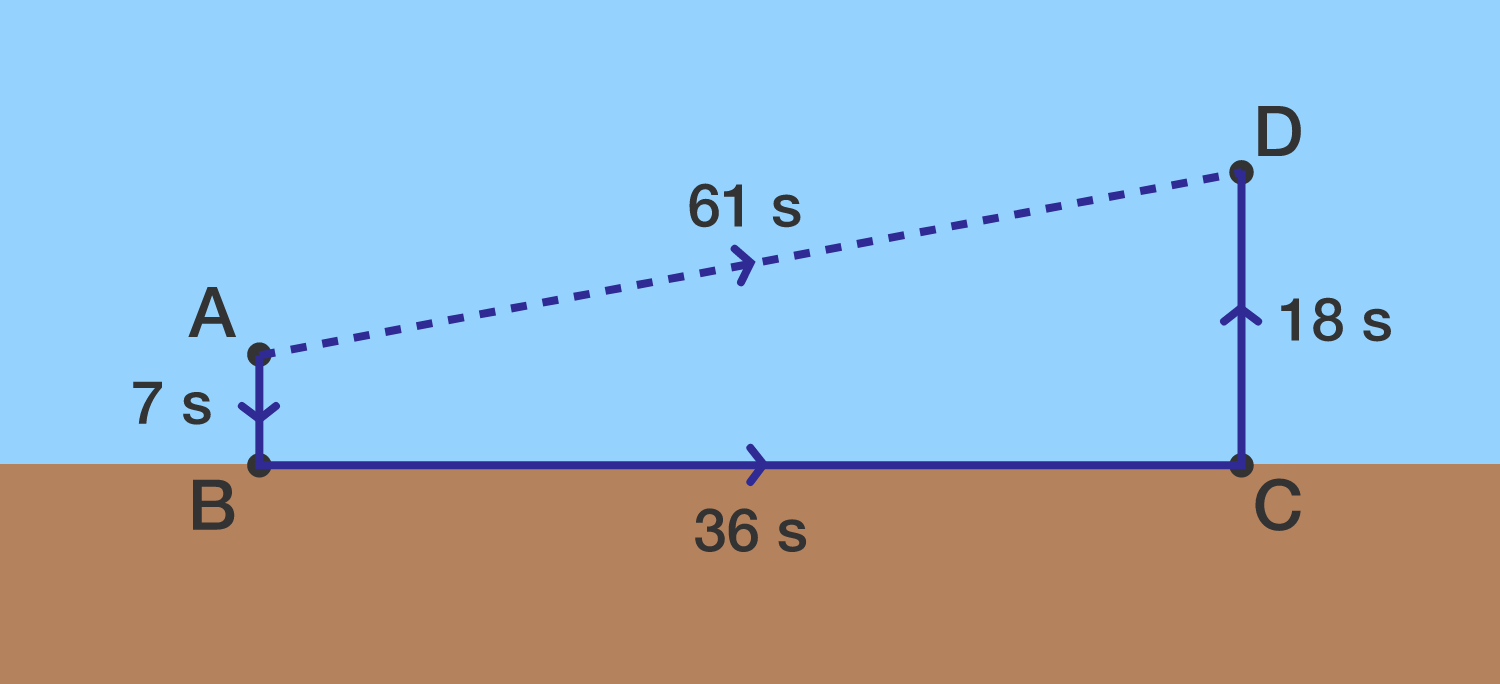Segments $\overline{AB}$ and $\overline{CD}$ are perpendicular to the shoreline.

Note: When Agatha swims to and from the shoreline, the angles don't have to be 90 degrees.

A two-state quantum system is called a qubit. A qubit can be realized by a photon which has two different polarization states $\ket{0^{\circ}}$ and $\ket{90^{\circ}}$ which correspond to polarization along the vertical and horizontal axes, respectively. The ability to precisely and reliably manipulate qubits is key to the advent of large-scale quantum computing

A linearly polarized photon aligned with angle $\theta$ can be split into its $\ket{0^{\circ}}$ and $\ket{90^{\circ}}$ components with amplitudes corresponding to the projection of the wave on the measurement axis. These states are orthogonal, so a pure $\ket{0^{\circ}}$ photon has no $\ket{90^{\circ}}$ component, and vice versa: $\ket{\theta ^ \circ} = \cos(\theta) \ket{0^{\circ}} + \cos(90 ^ \circ -\theta) \ket{90^{\circ}}, \quad \theta \in [0^\circ,360^\circ].$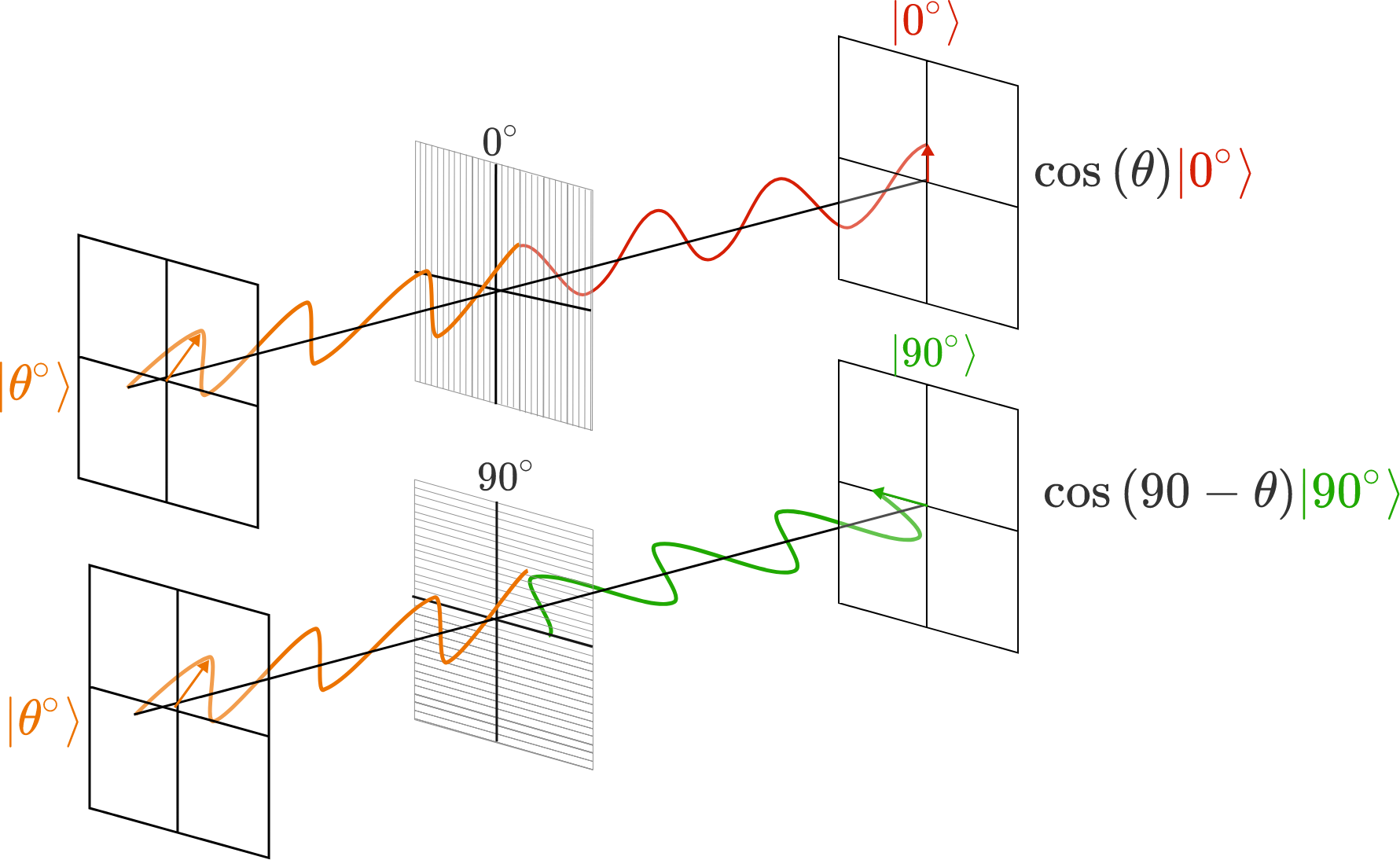One way to manipulate a photon qubit is with polarization filters. Each filter measures and absorbs all photons in the $\ket{\alpha+90 ^ \circ}$ state, while all others pass through. Consider a series of three linear polarization filters, each rotated by an angle of $\alpha = 30 ^ \circ, 60 ^ \circ,$ and $90 ^ \circ,$ respectively, with respect to the $\ket{0^{\circ}}$ polarization direction.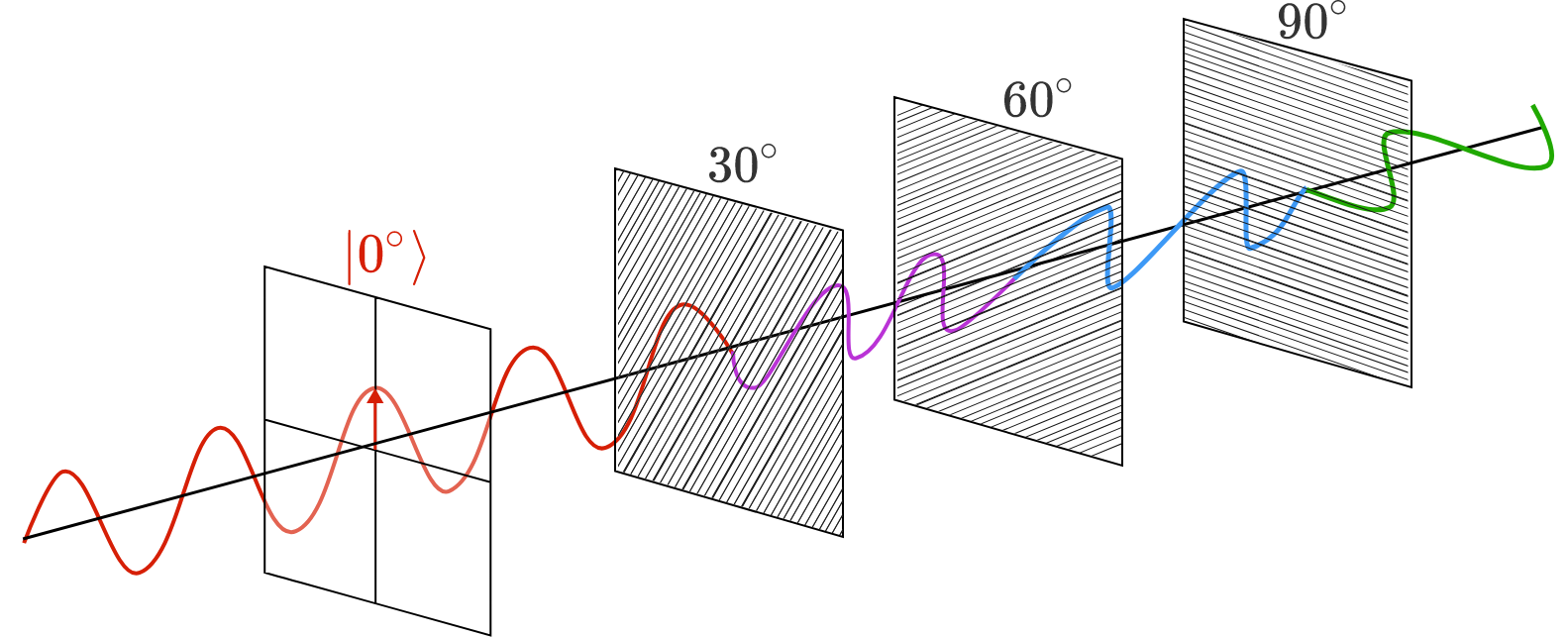What is the probability $P$ that a $\ket{0^{\circ}}$ photon passes all three polarizing filters and emerges as a $\ket{90^{\circ}}$ photon?

In a school, each student is assigned a positive factor of $60^{60}$, but no student's number is the greatest common divisor of any two student numbers (one of which may be their own).

What is the maximum number of students in this school?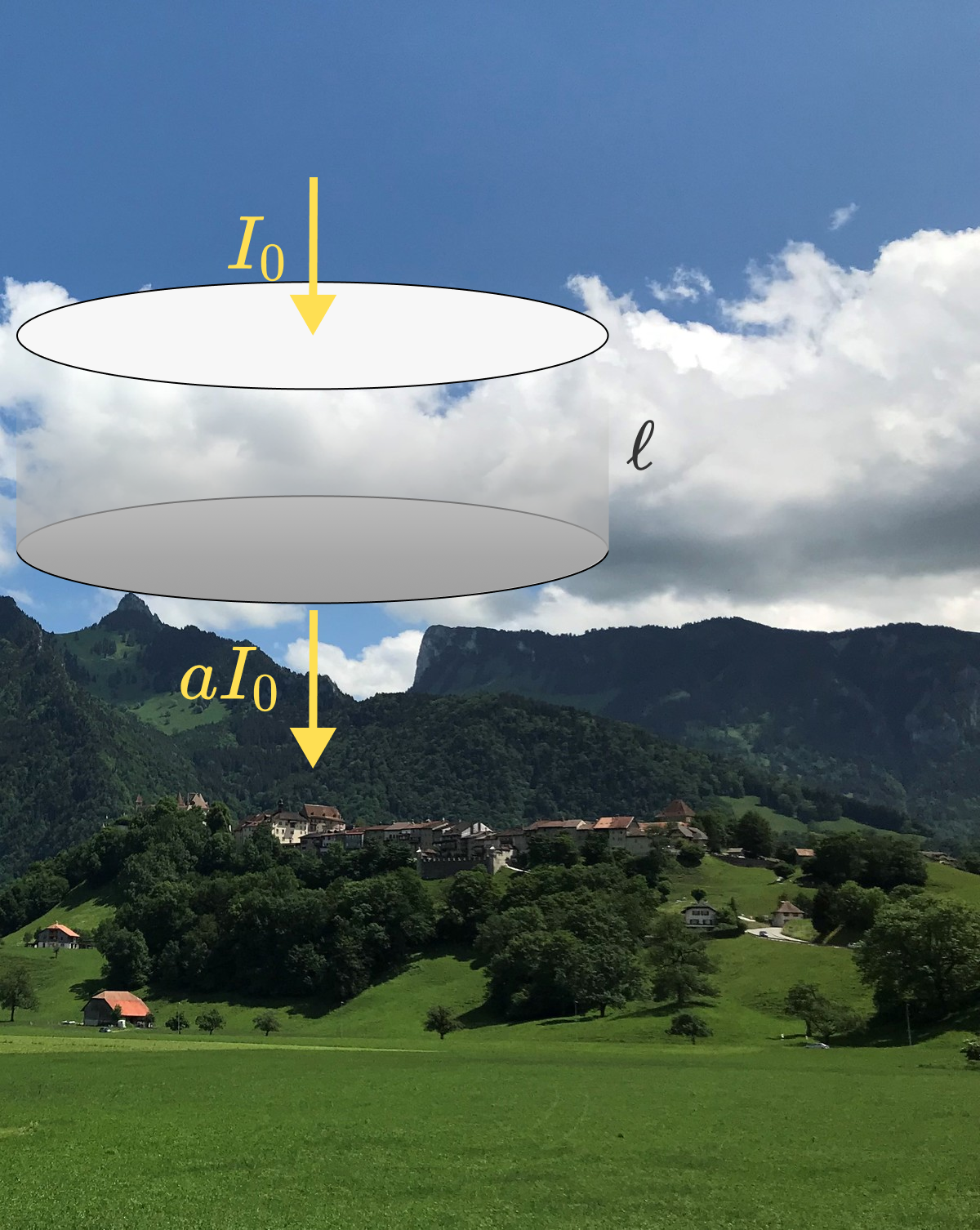Thinner clouds are white and shiny all over; thick clouds are shiny on the top and dark at the bottom. This effect is caused by incident sunlight scattering off of water droplets in the cloud. Since the sunlight is incident from the top, less light makes it all the way through the cloud to be scattered into our eyes, resulting in a darker bottom.

Suppose that the water droplets in the cloud are $\SI{20}{\micro\meter}$ in diameter and there are about $200$ droplets in a cubic centimeter of cloud material. Sunlight with intensity $I_0$ is incident from above the cloud, and when light hits a droplet, it is scattered in a random direction with no loss of energy.

What fraction $a$ of the incident sunlight is coming out at the bottom of a $\ell=100\text{ m}$-thick cloud in the form of scattered light? Give your answer rounded to three decimal places.

Hint: The scattering of light through a cloud is much like the scattering that occurs on ground level on a foggy day. You may also find it useful to try treating the propagation of the scattered light as a diffusion problem.

×